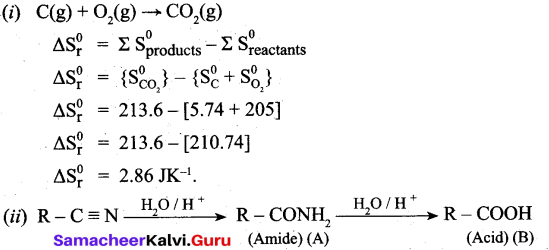# Tamil Nadu 11th Chemistry Previous Year Question Paper March 2019 English Medium

Students can Download Tamil Nadu 11th Chemistry Previous Year Question Paper March 2019 English Medium Pdf, Tamil Nadu 11th Chemistry Model Question Papers helps you to revise the complete Tamilnadu State Board New Syllabus and score more marks in your examinations.

## TN State Board 11th Chemistry Previous Year Question Paper March 2019 English MediumTime: 2½ Hours
Total Score: 90 Marks

General Instructions:

1. The question paper comprises of four parts.
2. You are to attempt all the parts. An internal choice of questions is provided wherever applicable.
3. All questions of Part I. II, III and IV are to be attempted separately.
4. Question numbers 1 to 15 in Part I are Multiple Choice Questions of one mark each. These are to be answered by choosing the most suitable answer from the given four alternatives and writing the option code and the corresponding answer.
5. Question numbers 16 to 24 in Part II are two-mark questions. These are to be answered in about one or two sentences.
6. Question numbers 25 to 33 in Part III are three-mark questions. These are to be answered in about three to five short sentences.
7. Question numbers 34 to 38 in Part IV are five-mark questions. These are to be answered in detail. Draw diagrams wherever necessary.Part- I

Answer all the Questions. [Answers are in Bold] [15 x 1 = 15]

Choose the most appropriate answer from the given four alternatives and write the option code and the corresponding answer.

Question 1.
Many of the organic compounds are inflammable because of its
(a) Vander Waal’s force
(b) Co-ordinate nature
(c) Covalent nature
(d) Ionic nature
(c) Covalent nature

Question 2.
When Ang is negative in chemical equilibrium reaction, then ..
(a) Kp < Kc
(b) Kp = 1 /Kc
(c) Kp = Kc (RT)-vc
(d) Kp > Kc
(c) Kp = Kc (RT)-vcQuestion 3.
Find A in the following equation.
$$\mathrm{CaO}+3 \mathrm{C} \stackrel{3273 \mathrm{K}}{\longrightarrow} \mathrm{A}+\mathrm{CO}$$
(a) CaC2
(b) CO2
(c) Ca
(d) Ca2O
(d) Ca2O

Question 4.
Splitting of spectral lines in an electric field is called
(a) Compton effect
(b) Stark effect
(c) Zeeman effect
(d) Shielding effect
(a) Compton effectQuestion 5.
Which of the following species does not exert a resonance effect?
(a) C6H5NH2
(b) C6H5NH3
(c) C6H5OH
(d) C6H5CI
(b) C6H5NH3

Question 6.
Match the following:
Compound – Uses
1. Chloro picrin (i) Detection of primary amine
2. Methyl Isocyanide (ii) DDT
3. Chloro benzene (iii) Paint remover
4. Methylene chloride (iv) Soil sterilizer
(a) 1- (iv), 2 – (iii), 3 – (ii), 4 – (i)
(b) 1- (Hi), 2 – (iv), 3 – (ii), 4 – (i)
(c) 1- (i), 2 – (ii), 3 – (iv), 4 – (iii)
(d) 1- (iv), 2 – (i), 3 – (ii), 4 – (iii)
(d) 1 – (iv), 2 – (i), 3 – (ii), 4 – (iii)

Question 7.
Use of hot air balloon in the meteorological observatory is an application of
(a) Kelvin’s Law
(b) Brown’s Law
(c) Boyle’s Law
(d) Newton’s Law
(b) Brown’s LawQuestion 8.
What is the pH of rain water?
(a) 5.6
(b) 4.6
(c) 6.5
(d) 7.5
(a) 5.6

Question 9.
Which compound is named as “Blue John” among the following compounds?
(a) Ca3(PO4)2
(b) CaO
(c) CaH2
(d) CaF2
(d) CaF2

Question 10.
The element with positive electron gain enthalpy is ………………………… .
(a) Argon
(b) Fluorine
(c) Hydrogen
(d) Sodium
(d) SodiumQuestion 11.
Which of the following mMecule does not contain n bond?
(a) CO2
(b) H2O
(c) SO2
(d) NO2
(c) SO2

Question 12.
Which of the following compound has same percentage of Carbon as that of ethylene (C2H4)?
(a) benzene
(b) ethane
(c) propene
(d) ethyne
(a) benzene

Question 13.
The SI unit of Molar heat capacity is
(a) JK-1 mol-1
(b) kJ mol+1
(c) kJ mol-1
(d) cm
(c) kJ mol-1

Question 14.
What percentage of solution of H202 is called as “100-Volume” H202?
(a) 15%
(b) 50%
(c) 20%
(d) 30%
(a) 15%Question 15.
Osmotic pressure (n) of a solution is given by the relation
(a) πRT = n
(b) V = πnRT
(c) π = nRT
(d) πV = nRT
(b) V = πnRT

Part-II

Answer any six of the following questions. Question no. 24 is compulsory. [6 x 2 = 12]

Question 16.
State and explain Pauli’s Exclusion Principle.
Pauli’s exclusion principle states that “No two electrons in an atom can have the same set of values of all four quantum numbers”.
Illustration: H(Z = 1) Is1.

One electron is present in hydrogen atom, the four quantum numbers are n = 1, l = 0, m = 0 and s = + ½. For helium Z = 2. He: Is2. In this one electron has the quantum number same as that of hydrogen, n = 1, l = 0 m = 0 and s = + ½. For other electron, fourth quantum number is different, i.e. n = 1, l = 0, m = 0 and s = – ½.

Question 17.
Define – Valency.
The valency of an element may be defined as the combining capacities of elements. The electrons present in the outermost shell are called valence electrons and these electrons determine the valency of the atom.Question 18.
What are ideal gases?

• A gas that follows Boyle’s law, Charles’ law and Avogadro law strictly is called an ideal gas. It is assumed that intermolecular forces are not present between the molecules of an ideal gas.
• Ideal gases are the gases that obey gas laws or gas equation PV = nRT.
• Real gases do not obey gas equation. PV = nRT.
• The deviation of real gases from ideal behaviour is measure in terms of a ratio of PV to PV nRT. This is termed as compression factor (Z). Z = $$\frac{P V}{n R T}$$

Question 19.
State the third law of Thermodynamics.
It states that the entropy of pure crystalline substance at absolute zero is zero, (or)

It can be stated as “it is impossible to lower the temperature of an object to absolute zero in a finite number of steps”. Mathematically $$\lim _{T \rightarrow 0} S=0$$Question 20.
What is called Bond Length? Name the techniques through which the length of a bond can be determined.
The distance between thS nuclei of two covalently bonded atoms is called bond length. For e.g„ in a covalent molecule A – B, the bond length is equal to the sum of the radii of bonded atoms, i.e., γA + γB = bond length.

The length of a bond can be determined by spectroscopic, x-ray diffraction and electron- diffraction techniques.

Question 21.
Describe the reaction involved in the detection of Nitrogen in an organic compound by Lassaigne Method.
Detection of Nitrogen: The following reactions are involved in the detection of nitrogen with formation of prussian blue precipitate conforming the presence of nitrogen in an organic compound.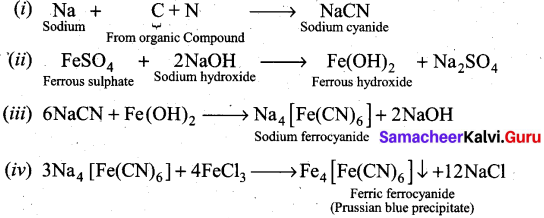Question 22.
How is Alkane prepared from Grignard reagent?
CH3MgX + HOH → CH4 + Mg(OH)X.
Water would protonate the grignard reagent and destroy the grignard reagent, because the grignard carbon atom is highly nucleophilic. This would form a hydrocarbon. Therefore to make a grignard solution, only ether is the best solvent and water or alcohol are not used for that purpose.

Question 23.
Define – Acid rain.
Rain water has a pH of 5.6 due to the dissolution of CO2 into it. Oxides of sulphur and nitrogen in the atmosphere may be absorbed by droplets of water that make up the clouds and get chemically converted into sulphuric acid and nitric acid. Due to this the pH of rain water drops below the level of 5.6. Hence it is called acid rain.

Question 24.
Which is the suitable method for detection of Nitrogen present in food and fertilizers?
Kjeldahl’s method is the suitable for detection of nitrogen present in food and fertilizers.Part-III

Answer any six of the following questions. Question No. 33 is compulsory. [6 x 3 = 18]

Question 25.
Calculate the equivalent mass of H2SO4.
Sulphuric acid = H2SO4
Molar mass of Sulphuric acid = 2 + 32 + 64 = 98
Basicity of Sulphuric acid = 2
Equivalent mass of acid =
$$=\frac{\text { Molar mass of an acid }}{\text { Basicity }}$$
$$=\frac{98}{2}=49 \mathrm{geq}^{-1}$$

Question 26.
Explain diagonal relationship.

• On moving diagonally across the periodic table, the second and the third period elements show certain similarities.
• Even though the similarity is not same as we see in a group, it is quite pronounced in the following pair of elements.
•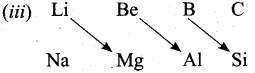• The similarity in properties existing between the diagonally placed elements is called “diagonal relationship”.Question 27.
How is Tritium prepared?
It occurs naturally as a result of nuclear reactions induced by cosmic rays in the upper atmosphere.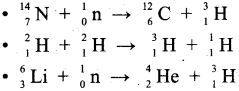Question 28.
Define – Le-Chatelier principle.
It states that “If a system at equilibrium is disturbed, then the system shifts itself in a direction that nullifies the effect of that disturbance.

Question 29.
State the term “Isotonic solution”.

1. Two solutions having same osmotic pressure at a given temperature are called isotonic solutions.
2. When such solutions are separated by a semipermeable membrane, solvent flow between one to the other on either direction is same, i.e., the net solvent flow between two isotonic solutions is zero.Question 30.
Both C2H2 and CO2 have the same structure. Explain why.
H – C = C – H and O = C = O
Both has,
Linear- Molecular geometry
Zero – Dipole moment
sp – Hybridization of C in C2H2 and CO2. Hence C2H2 and CO2 have the same structure.

Question 31.
Write note on Williamson’s Synthesis.
Williamson’s synthesis: Haloalkanes, when boiled with sodium alkoxide, gives the corresponding ether.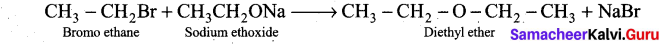Question 32.
Explain why Ca(OH)2 is used in white washing.
(i) Ca(OH)2 used in white washing, this is due to disinfectant nature.
(if) Calcium hydroxide reacts with CO2 in air to form thin layer of calcium carbonate on the walls. It gives a shiny finish to the walls.Question 33.
Give the structural formula for the following compounds.
(a) m – dinitrobenzene
(b) p – dichlorobenzene
(c) 1,3, 5, Tri-methyl Benzene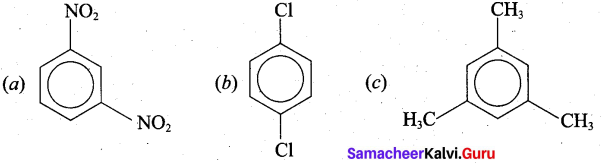Part – IV

Question 34.
(a) (i) Calculate oxidation number of oxygen in H2O2.
(ii) Write the de-Broglie equation.
[OR]
(b) (i) State and explain Dobereiner’s “Triad”.
(ii) Complete the following equation.
(a)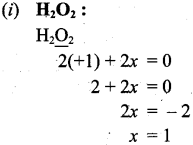Therefore oxidation number of ‘O’ in H2O2 is -1.(ii) 1. Louis de Broglie extended the concept of dual nature of light to all forms of matter. To quantify this relation, he derived an equation for the wavelength of a matter wave.
2. He combined the following two equations of energy of which one represents wave character (ho) and the other represents the particle nature (mc2).
Planck’s quantum hypothesis:
E = hv …………………………. (1)
Einsteins mass-energy relationship:
E = mc2 ………………………….. (2)
From (1) and (2)
hv = mc2
h/λ = mc2
∴ λ = $$\frac{h}{m c}$$ ……………………………….. (3)

The equation (3) represents the wavelength of photons whose momentum is given by. me. (Photons have zero rest mass).

3. For a particle of matter with mass m and moving with a velocity v, the equation (3) can be written as λ = $$\frac{h}{m c}$$ ……………………………….. (3)

[OR](b) (i) Johann Dobereiner noted that elements with similar properties occur in groups of three which he called triads. It was seen that invariably, the atomic weight of the middle number of the triad was nearly equal to the arithmetic mean of the weights of the other two numbers of the triad. For e.g.,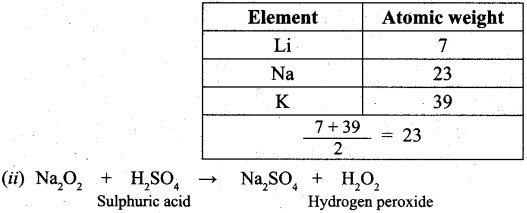Question 35.
(a) (i) Among the alkaline earth metals BeO is insoluble in water but other oxides are soluble. Why?
(ii) State Diffusion Law.
[OR]
(b) (i) Calculate the entropy change during the melting of one mole of ice into water at 0°C. Enthalpy of fusion of ice is 6008 J mol-1.
(ii) Write the balanced chemical equation for Kc = $$\frac{\left[\mathbf{C a O}_{(\mathbf{s})}\right]\left[\mathbf{C O}_{2(\mathbf{g})}\right]}{\left[\mathbf{C a C O}_{3(\mathbf{(})}\right]}$$
(a) (i) Lattice energy of BeO is comparatively higher than the hydrogen energy. Therefore it is almost insoluble in water. Whereas other alkaline earth metals oxides have high hydration energy. Hence they are soluble in water.(ii) Diffusion is the spreading of molecules of a substance throughout a space or a second substance. Diffusion refers to the ability of the gases to mix with each other. E.g., Spreading of something such as brown tea liquid spreading through the water in a tea cup.

[OR]

(b)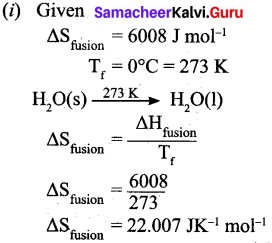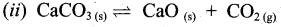Question 36.
(a) (i) NH3 and HC1 do not obey Henry’s law. Why?
(ii) Write the structure of the following compunds. (A) NH3 (B) BF3

[OR]

(b) (i) Identify the cis and trans isomers for the following compounds.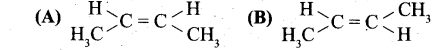(ii) Explain with example the Positive Mesomeric Effect.
(a) (i) Only the less soluble gases obeys Henry’s law. NH3 and HC1 react with (or soluble) water and hence does not obey Henry’s law.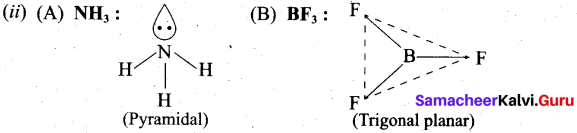(b)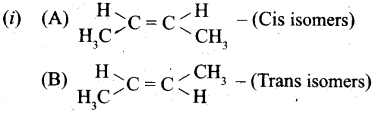(ii)

1. Positive mesomeric effect occurs, when the electrons move away from substituent attached to the conjugated system.
2. It occurs, if the electron releasing substituents are attached to the conjugated system.
3. The attached group has a tendency to release electrons through resonance, these electron releasing groups are usually denoted as +R or +M groups
4. Examples: —OH, —SH, —OR, —SR, —NH2 etc.

Question 37.
(a) (i) Write the IUPAC name for the following compound.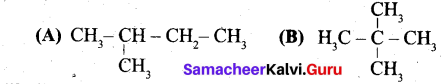(ii) What are Nucleophiles and Electrophiles? Give one example each.

[OR]

(b) (i) How will you get the following products with the given reactants?
(A) Acetylene → Benzene
(B) Phenol → Benzene
(C) Benzene → Toluene
(ii) Write any two different components you get during fractional distillation of Coal Tar at any two different temperatures.
(a)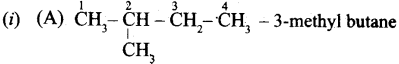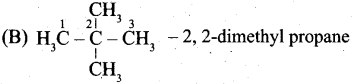(ii) Electrophiles: Electrophiles are reagents that are attracted towards negative charge or electron rich center. They are either positively charged ions or electron deficient neutral molecules.
Example, CO2, AlCl3, BF3, FeCl3, N0+, NO2+, etc.

Nucleophiles: Nucleophiles are reagents that has high affinity for electropositive centers. They possess an atom has a unshared pair of electrons. They are usually negatively charged ions or electron rich neutral molecules.
Example: NH3, R-NH2, R-SH, H2O, R-OH, CN, OH etc.

[OR]

(b)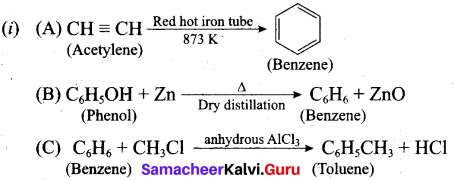(ii) Coal tar is a viscous liquid obtained by the pyrolysis of coal. During fractional distillation, coal tar is heated and distills away its volatile compounds, namely, benzene, toluene, xylene in the temperature range of 350 K to 443 K. These vapours are. collected at the upper part of the fractionating column.

 Name of the fraction Temperature Range Name of the component Crude light oil 350- 443 K Benzene, Toluene, Xylene Middle oil 443 – 503 K Phenol, Naphthalene Heavy oil 503 – 543 K Naphthalene, Cresol Green oil 543 – 633 K Anthracene Pitch oil 633 K Residue

Question 38.
(a) (i) A compound having the empirical formula C6H6O has the vapour density 47. Find its Molecular formula.
(ii) The Simple Aromatic Hydrocarbon compound (A) reacts with Bromine to give (B). Compound (A) reacts with Raney Ni and gives (C). Identify (A), (B) and (C).[OR]

(b) (i) C(s) + O2(g) → CO2(g)
Calculate the standard entropy change for the above reaction. Given the standard entropies of CO(s) + C(s), O2(g) are 213.6, 5.740 and 205 JK-1 respectively.
(ii) Identify the compound (A) and (B).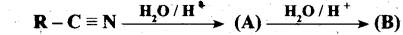(a) (i) Empirical formula = C6H6O
Empirical formula mass
= (6 x 12) + (6 x 1) + (16 x 1)
= 72 + 6+16
= 94
Vapour density =47
Molecular mass = 2 x Vapour density
= 2 x 47
= 94
Molecular formula = n x Empirical formula
$$\mathrm{n}=\frac{\text { Molecular mass }}{\text { Empirical formula mass }}$$
n = 94/94 ⇒ n = 1
Molecular formula = 1 x C6H6O
= c6H6O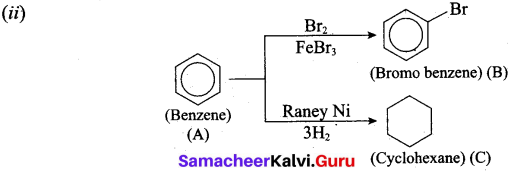[OR]